# 手把手：用Python搭建机器学习模型预测黄金价格

+关注继续查看

GLD 是最大的以黄金进行直接投资的ETF交易基金。

• 导入Python库并读取黄金ETF 的数据
• 定义解释变量
• 将数据切分为模型训练数据集和测试数据集
• 建立线性回归模型
• 预测黄金ETF的价格

# LinearRegression is a machine learning library for linear regression

from sklearn.linear_model import LinearRegression

# pandas and numpy are used for data manipulation

import pandas as pd

import numpy as np

# matplotlib and seaborn are used for plotting graphs

import matplotlib.pyplot as plt

import seaborn

# fix_yahoo_finance is used to fetch data import fix_yahoo_finance as yf

# Read data

# Only keep close columns

Df=Df[['Close']]

# Drop rows with missing values

Df= Df.dropna()

# Plot the closing price of GLD

Df.Close.plot(figsize=(10,5))

plt.ylabel("Gold ETF Prices")

plt.show()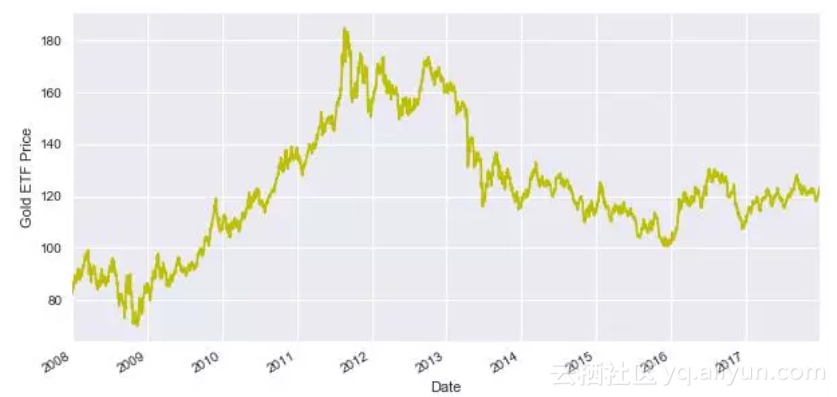Df['S_3'] = Df['Close'].shift(1).rolling(window=3).mean()

Df['S_9']= Df['Close'].shift(1).rolling(window=9).mean()

Df= Df.dropna()

X = Df[['S_3','S_9']]

X.head()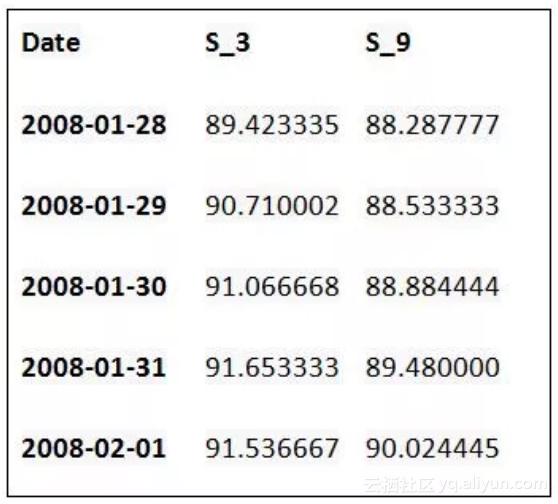y = Df['Close']

y.head()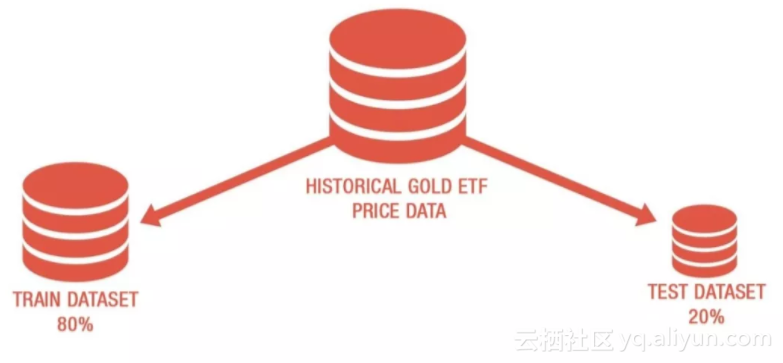• 前80%的数据用于训练模型，其余的数据用来测试模型。
• X_train 和y_train是训练数据集。
• X_test & y_test是测试数据集。
t=.8

t = int(t*len(Df))

# Train dataset

X_train = X[:t]

y_train = y[:t]

# Test dataset

X_test = X[t:]

y_test = y[t:]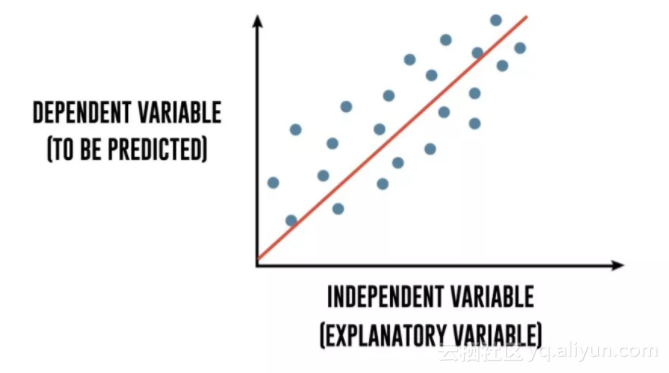Y = m1 * X1 + m2 * X2 + CGold ETF price = m1 * 3 days moving average + m2 * 15 days moving average + c

linear = LinearRegression().fit(X_train,y_train)

print "Gold ETF Price =", round(linear.coef_,2), \

"* 3 Days Moving Average", round(linear.coef_,2), \

"* 9 Days Moving Average +", round(linear.intercept_,2)

predicted_price = linear.predict(X_test)

predicted_price = pd.DataFrame(predicted_price,index=y_test.index,columns = ['price'])

predicted_price.plot(figsize=(10,5))

y_test.plot()

plt.legend(['predicted_price','actual_price'])

plt.ylabel("Gold ETF Price")

plt.show()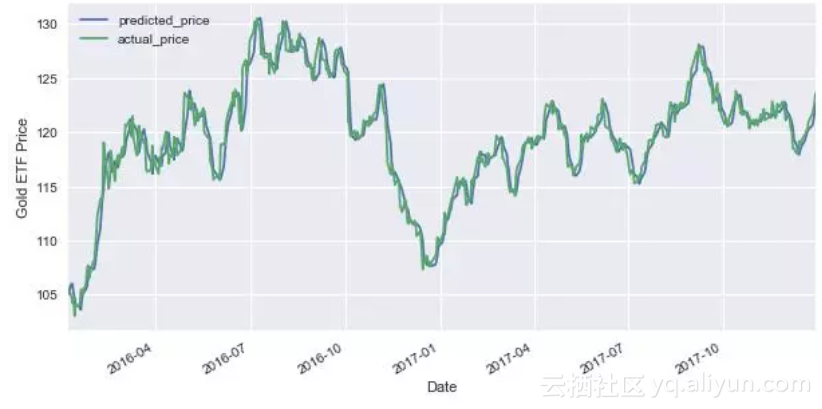r2_score = linear.score(X[t:],y[t:])*100

float("{0:.2f}".format(r2_score))|
4月前
|

【机器学习实战项目】10分钟学会Python怎么用LDA线性判别模型进行分类预测（五）
【机器学习实战项目】10分钟学会Python怎么用LDA线性判别模型进行分类预测（五）
80 0
|
4月前
|

【机器学习实战项目】10分钟学会Python怎么用支持向量机SVM进行分类预测（三）
【机器学习实战项目】10分钟学会Python怎么用支持向量机SVM进行分类预测（三）
66 0
|
4月前
|

【机器学习实战项目】10分钟学会Python怎么用朴素贝叶斯Bayes进行分类预测（二）
【机器学习实战项目】10分钟学会Python怎么用朴素贝叶斯Bayes进行分类预测（二）
65 0
|
4月前
|

【机器学习实战项目】10分钟学会Python怎么用Logistic回归进行分类预测（一）
【机器学习实战项目】10分钟学会Python怎么用Logistic回归进行分类预测（一）
56 0
|
4月前
|

2021-4月 Python机器学习——名词概念学习，概念解释
2021-4月 Python机器学习——名词概念学习，概念解释
75 0
|
4月前
|

2021-4月Python 机器学习——中文新闻文本标题分类
2021-4月Python 机器学习——中文新闻文本标题分类
60 0
|
4月前
|

2021-4月Python 机器学习——中文新闻文本标题分类(简单容易版)
2021-4月Python 机器学习——中文新闻文本标题分类(简单容易版)
107 0
|
4月前
|

Python机器学习——决策树
Python机器学习——决策树
53 0
|
5月前
|

【数学建模】机器学习预测算法（KNN、随机森林、网格搜索对决策树、支持向量机）（Python代码实现）【2021华为杯】
【数学建模】机器学习预测算法（KNN、随机森林、网格搜索对决策树、支持向量机）（Python代码实现）【2021华为杯】
117 0